# Fluid

We have vessels containing 7 liters, 5 liters and 2 liters. Largest container is filled with fluid the others empty. Can you only by pouring get 5 liters and two 1 liter of fluid? How many pouring is needed?

x =  6

### Step-by-step explanation:

$x=6=6$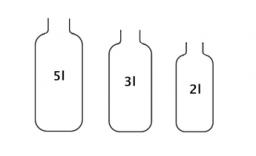Did you find an error or inaccuracy? Feel free to write us. Thank you!Tips to related online calculators
Tip: Our volume units converter will help you with the conversion of volume units.

## Related math problems and questions:

• Alarm clockThe old watchmaker has a unique digital alarm in its collection that rings whenever the sum of digits of the alarm is equal to 21. Find out when the alarm clock will ring. What is their number? List all options . ..
• MilkThere were 22 liters of milk in three containers. There was 6 liters more in the first container than in the second. After pouring 5 liters from the first container into the third container, the same quantity of milk is in the second and third container.
• BicyclesYou're the owner of the transport 's learning playground. Buy bicycles of two colors but you've got to spend accurately 120000 Kč. Blue bike costs 3600Kč and red bicycle 3200Kč.
• Equation algebraogram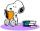Solve the equation: oco + ivo = cita How much has the task of solutions?
• All pairs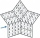Determine all pairs (m, n) of natural numbers for which is true: m s (n) = n s (m) = 70, where s (a) denotes the digit sum of the natural number a.
• Twos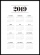Vojta started writing the number of this year 2019202020192020 into the workbook. .. And so he kept going. When he wrote 2020 digits, he no longer enjoyed it. How many twos did he write?
• Star equationWrite digits instead of stars so that the sum of the written digits is odd and is true equality: 42 · ∗8 = 2 ∗∗∗
• Bucket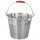How many 5-liter buckets do you have to pour into a 0.2 m3 container to make it full?
• Water tanksA different amount of water is poured into each of the three identical vessels. In the first vessel, water fills 30% of its volume and in the second vessel 40% of its volume. The third container contains 19 liters of water. If we distributed the water fro
• MO-Z5-3-66 tilesThe picture shows a square tiles with side 10 dm which is composed of four identical small rectangles and squares. Circumference of small square is five times smaller than the circumference of the entire tile. Determine the dimensions of the rectangle.
• Glasses of juice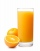Jake’s mom filled a 2-liter container with juice. Then Jake poured 2 glasses of juice. Each glass had exactly 400 milliliters of juice. How many liters of juice remain in the container?
• 3 cats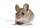3 cats eat 3 mice in 3 days. How many mice will be eaten by 10 cats in 10 days?
• Container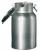How many three-quarters liters jar fill 30 liters container?
• Water containerThe cube-shaped container is filled to two-thirds of its height. If we pour 18 liters, it will be filled to three-fifths of the height. What is the volume of the whole container?
• Soda factory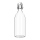In the soda factory, they produced 50 hl of lemonade, which they filled into 2 l bottles. How many bottles did they fill?
• Mineral water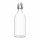The bottle contains 1.5 liters of mineral water. Pour all the water from the bottle into empty cups with a volume of 1/3 l. All but one will be filled to the brim. What part of the volume of the last cup is filled with water?Add marks (+, -, *, /, brackets) to fullfill equations 1 3 6 5 = 10 This is for the 4th grade of the primary school - with no negative numbers yet